# How to use the Excel TIME function

The TIME function returns the time in time format. It takes three integers arguments representing hours, minutes & seconds respectively.
Syntax:

=TIME (hours, min, seconds)

Hour: hour cycle (24 hrs)
Minutes: minute cycle (60 min)
Seconds: second cycle (60 sec)

For example: =TIME(1, 1, 1) returns the 1:01 PM; remember to change the format of the cell from hh:mm AM/PM to hh:mm:ss AM/PM as shown in the snapshot.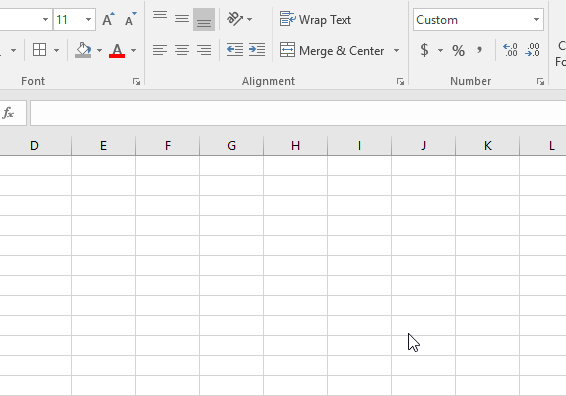Let’s understand this function using it an example.

Here we have some time values and it needed to be represented in Time format.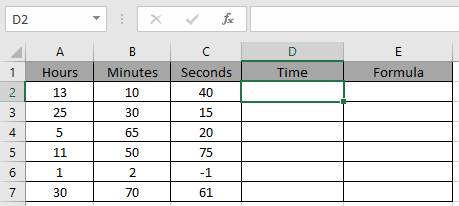Use the formula

=TIME(A2,B2,C2)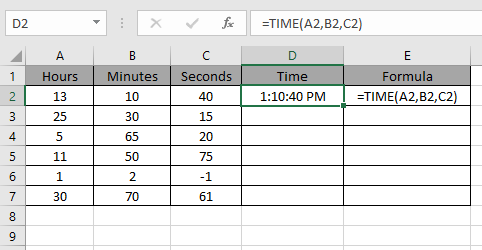You don’t need to remember the hr, min or sec cycle. Excel Time function takes the input as integers and if any integer crosses the cycle range gets divided by its unit cycle and returns remainder as shown below examples.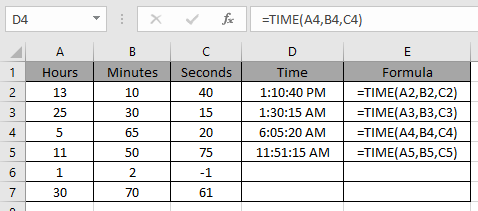If seconds cycle completes the quotient is added to minutes and same goes for minute cycle and hour cycle.

If an integer is negative in seconds as an argument then it gets subtracted from minutes time and same goes for minutes and hours.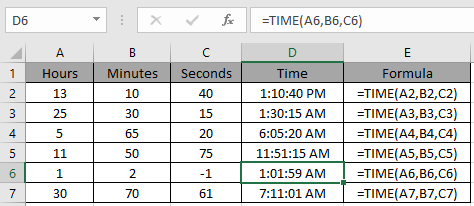As you can see we got the time value in time format.

Hope you understood how to use TIME function and referring cell in Excel. Explore more articles on Excel Date and Time function here. Please feel free to state your query or feedback for the above article.

Related Articles:

How to use the NOW Function in Excel

How to Use TODAY Function in Excel

How to use WEEKDAY Function in Excel

How to use the MONTH Function in Excel

How to Use the YEAR Function in Excel

Popular Articles:

How to use the VLOOKUP Function in Excel

How to use the COUNTIF function in Excel 2016

How to Use SUMIF Function in Excel

Terms and Conditions of use

The applications/code on this site are distributed as is and without warranties or liability. In no event shall the owner of the copyrights, or the authors of the applications/code be liable for any loss of profit, any problems or any damage resulting from the use or evaluation of the applications/code.